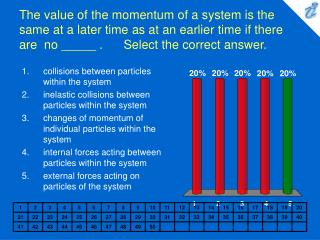DownloadDownload PresentationThe value of the momentum of a system is the same at a later time as at an earlier time if there are no _____ . Se

# The value of the momentum of a system is the same at a later time as at an earlier time if there are no _____ . Se

Download Presentation## The value of the momentum of a system is the same at a later time as at an earlier time if there are no _____ . Se

- - - - - - - - - - - - - - - - - - - - - - - - - - - E N D - - - - - - - - - - - - - - - - - - - - - - - - - - -
##### Presentation Transcript

1. The value of the momentum of a system is the same at a later time as at an earlier time if there are no _____ . Select the correct answer. • collisions between particles within the system • inelastic collisions between particles within the system • changes of momentum of individual particles within the system • internal forces acting between particles within the system • external forces acting on particles of the system

2. Two bodies with masses m1 and m2 are both moving east with velocities of magnitudes v1 and v2, where v1 is less than v2. The magnitude of the velocity of this two-body system's center of mass is _____ . Select the correct answer. • less than v1 • equal to v1 • equal to the average of v1 and v2 • greater than v1 and less than v2 • greater than v2

3. A rocket with an initial mass of 1500 kg adjusts its thrust by varying the rate at which mass is ejected. The ejection speed relative to the rocket is 40 km/s. If the acceleration of the rocket is to have a magnitude of 20 m/s2 at an instant when its mass is 80% of the original mass, at what rate is mass being ejected at that instant? Ignore any external forces on the rocket. Select the correct answer. • 0.60 kg/s • 0.70 kg/s • 0.40 kg/s • 0.30 kg/s • 0.20 kg/s

4. A 3.0-kg object moving in the positive x direction has a one-dimensional elastic collision with a 9.0-kg object initially at rest. After the collision the 9.0-kg object has a velocity of 2.0 m/s in the positive x direction. What was the initial speed of the 3.0 kg object? Select the correct answer. • 4.0 m/s • 3.6 m/s • 4.8 m/s • 2.8 m/s • 2.4 m/s

5. If you know the impulse that has acted on a body of mass m you can calculate _____ . Select the correct answer. • its initial velocity • its final velocity • its final momentum • the change in its velocity • its acceleration during the impulse

6. A 6.0-kg object moving 2.0 m/s in the positive x direction has a one-dimensional elastic collision with a 4.0-kg object moving 4.0 m/s in the opposite direction. What is the total kinetic energy of the two-mass system after the collision? Select the correct answer. • 44 J • 48 J • 35 J • 57 J • 26 J

7. A 3.0-kg object moving with a speed of 4.7 m/s collides perpendicularly with a wall and emerges with a speed of 3.0 m/s in the opposite direction. If the object is in contact with the wall for 3.1 m/s, what is the magnitude of the average force on the object by the wall? Select the correct answer. • 7.4 kN • 8.1 kN • 8.9 kN • 9.6 kN • 1.1 kN

8. A 7.0-kg object moving 5.0 m/s strikes a 5.0-kg object initially at rest. Immediately after the collision, the 7.0-kg object has a velocity of 3.0 m/s directed 60° from its initial direction of motion. What is the x-component of the velocity of the 5.0-kg object just after the collision? Select the correct answer. • 4.9 m/s • 5.9 m/s • 6.9 m/s • 7.8 m/s • 8.8 m/s

9. An 6.0-kg object moving 7.0 m/s in the positive x direction has a one-dimensional collision with a 4.0-kg object moving 3.0 m/s in the opposite direction. The final velocity of the 6.0-kg object is 2.0 m/s in the positive x direction. What is the total kinetic energy of the two-mass system after the collision? Select the correct answer. • 53 J • 42 J • 32 J • 85 J • 95 J

10. A 3.00 kg stone is dropped from a 26.1 m high building. When the stone has fallen 19.6 m, the magnitude of the impulse it has received from the gravitational force is _____ . Select the correct answer. • 9.8 N·s • 19.6 N·s • 29.4 N·s • 58.8 N·s • 117.6 N·s

11. A 2.0-kg object moving 8.0 m/s collides with and sticks to an 6.0-kg object initially at rest. Determine the kinetic energy lost by the system as a result of this collision. Select the correct answer. • 48 J • 58 J • 29 J • 19 J • 6.0 J